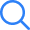# NCERT Solutions For Class 10 MathsStudents preparing for the class 10th board exam can refer to the NCERT Solutions for class 10 maths. It consists of step by step solutions of maths questions given in the NCERT Class 10th Maths textbook. These solutions are developed by the subject matter experts of Selfstudys in two languages: Hindi & English. It will boost the students' board exam preparations very easily. Because the Class 10 Maths NCERT Solutions that are available here are developed according to the CBSE Class 10th Syllabus 2022-23. Therefore, on this page students will be able to access the term wise NCERT class 10 maths solutions.

## NCERT Solutions for Class 10 Maths Chapter-Wise Exercises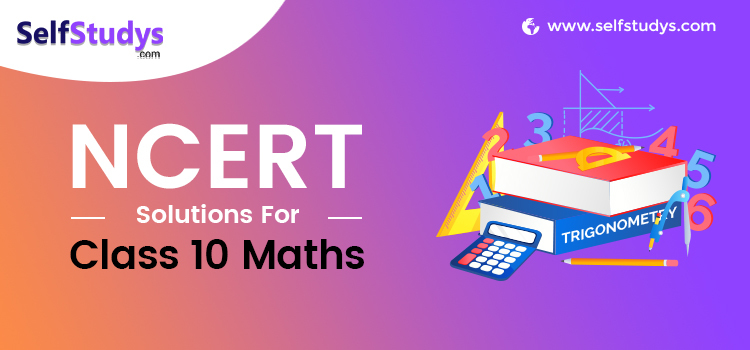These are extremely helpful and available here for free of cost. You can download the NCERT solutions for class 10 maths pdf as per your need. Also, you can access NCERT solutions for class 10 maths in Hindi

### Class 10 Maths NCERT SolutionsIn Class 10th there are a total of 15 chapters in maths, starting from the Real Numbers and ending at Probability. In order to pass the board exams, you must score at least 33 marks in mathematics. Hence, having a rigorous practice of std tenth mathematics becomes necessary. Therefore, we are providing the Class 10 Maths NCERT Solutions all chapters, topics, and exercises.

These are available in a simple and precise manner that boosts the efficacy of learning. The solutions are very easy to comprehend and can be used to solve even the complex topics too. While Preparing for the board examination and practicing the mathematics questions, you can refer to these solutions to ease your preparation process.

These CBSE NCERT Class 10 Maths Solutions can also be referred to by the other board’s students. It will help them to have a deep understanding of mathematical concepts.

### NCERT Solutions for Class 10 Maths Chapter-WiseNCERT Solutions Class 10 Maths Chapter-Wise gives students the facility to access the solved questions of all the chapters. These solutions follow the latest CBSE Term Wise Syllabus 2021-22. Therefore, you can get the class 10 maths solutions chapter-wise PDF format for both the terms. These will ease the process of comprehending the formulas, theorems and topics that are given in all the chapters of NCERT class 10 maths.

NCERT Solutions Class 10 Maths Chapters are as follows: Real Numbers, Polynomials, Pair of Linear Equations in Two Variables, Quadratic Equations, Arithmetic Progressions, Triangles, Coordinate Geometry, Introduction to Trigonometry, Some Applications of Trigonometry, Circles, Constructions, Areas Related to Circles, Surface Areas and Volumes, Statistics, Probability. The step by step solutions for these chapters can be downloaded here for free of cost in PDF File format also NCERT solutions for class 10 maths in Hindi medium

 NCERT Class 10 Maths Chapter Wise Solutions Chapter 1 Real Numbers Chapter 2 Polynomials Chapter 3 Pair of Linear Equations in Two Variables Chapter 4 Quadratic Equations Chapter 5 Arithmetic Progressions Chapter 6 Triangles Chapter 7 Coordinate Geometry Chapter 8 Introduction to Trigonometry Chapter 9 Some Applications of Trigonometry Chapter 10 Circles Chapter 11 Constructions Chapter 12 Areas Related to Circles Chapter 13 Surface Areas and Volumes Chapter 14 Statistics Chapter 15 Probability

### NCERT Solutions for Class 10 Maths Chapter 1 Real Numbers

Class 10 Maths Chapter 1 Real Numbers introduces students about the numbers that exist in this real world. Any number that you can think of is known as real numbers except for the complex numbers or those who are neither rational nor irrational. It is one of the fundamental topics that becomes the base of maths. Having a good knowledge about real numbers helps in scoring good marks in the board exams and in the upcoming classes (For those who choose mathematics to study in class 11th and 12th).

The Natural Numbers, Whole Numbers, Integers, Rational Numbers, Irrational Numbers, etc. are the topics on which the questions are given in this chapter. Our Class 10th Maths NCERT Solutions helps in solving these types of questions very efficiently. It is available here for free of cost to download and practice for the upcoming board exams.

#### Class 10 Maths NCERT Solutions Chapter 1 Real Numbers Exercises

Other CBSE study materials For Chapter 1 Real Numbers

NCERT & Other CBSE study materials For Chapter 1 Real Numbers are given here. There are various study resources for students that can help them to grasp the knowledge of chapter 1 to score good marks in the Xth Board Exam.

### NCERT Solutions for Class 10 Maths Chapter 2 Polynomials

NCERT Solutions Class 10 Maths Chapter 2 Polynomials is an expression of variables that involves addition, subtraction, multiplication, etc. There are different types of polynomials that have been discussed and explained in detail. This chapter begins with the definition of degree of the polynomial, linear polynomial, quadratic polynomial and cubic polynomial.

You can find the questions in this chapter related to these topics. In order to understand the polynomial's core concepts, you will have to do extensive practice. Therefore, for your support in learning, we have provided the NCERT Class 10 Maths Solutions pdf.

#### Class 10 Maths NCERT Solutions Chapter 2 Polynomials Exercises

Other CBSE study materials For Chapter 2 Polynomials

NCERT & Other CBSE study materials For Chapter 2 Polynomials are given here. There are various study resources for students that can help them to grasp the knowledge of chapter 2 to score good marks in the Xth Board Exam.

### NCERT Solutions for Class 10 Maths Chapter 3 Pair of Linear Equations in Two Variables

NCERT Solutions Class 10 Maths Chapter 3 Pair of Linear Equations in Two Variables comprises a total of 7 exercises. Each chapter consists of a variety of topics and its respective questions. Those who will solve those given questions will gain good control over this chapter. There are a few methods that have been used throughout this chapter, a few of them are Algebraic Method, Elimination Method, Cross-Multiplication Method, Substitution Method, etc.

Here you can get answers to all those questions that are explained explicitly in step by step manner. You will be able to get all those solutions in PDF file format.

#### Class 10 Maths NCERT Solutions Chapter 3 Pair of Linear Equations in Two Variables Exercises

Other CBSE study materials For Chapter 3 Pair of Linear Equations in Two Variables

NCERT & Other CBSE study materials For Chapter 3 Pair of Linear Equations in Two Variables are given here. There are various study resources for students that can help them to grasp the knowledge of chapter 3 to score good marks in the Xth Board Exam.

### Class 10 Maths NCERT Solutions Chapter 4 Quadratic Equations

NCERT Solutions Class 10 Maths Chapter 4 Quadratic Equations help students to know about the standard form of quadratic equations, solutions of quadratic equations by factorisation and by using the formula of quadratic and other more things are there to learn.

While being in the learning quadratic equation you will need to practice as much as possible. Meanwhile, you may be stuck at some questions and at that time the NCERT solutions class 10 maths chapter 4 that we are providing will be useful. It will provide hand-holding support and let you solve all kinds of problems given in the Quadratic Equations.

#### NCERT Solutions For Class 10 Maths Chapter 4 Quadratic Equations Exercises

Other CBSE study materials For Chapter 4 Quadratic Equations

NCERT & Other CBSE study materials For Chapter 4 Quadratic Equations are given here. There are various study resources for students that can help them to grasp the knowledge of chapter 4 to score good marks in the Xth Board Exam.

### NCERT Solutions for Class 10 Maths Chapter 5 Arithmetic Progressions

NCERT Solutions Class 10 Maths Chapter 5 Arithmetic Progressions is an interesting chapter. It is introduced in this chapter relating to represent the series or numbers in the nth term. Also, it helps in determining whether a series is in AP ( Arithmetic Progressions) or not. This is oriented in finding out the nth terms of different positions, sometimes it could be the first or sometimes it could be the last.

In order to solve all the questions given in the Arithmetic Progressions topics, you can take the help of the NCERT maths class 10 solutions for Arithmetic Progression.

#### Class 10 Maths NCERT Solutions Chapter 5 Arithmetic Progressions Exercises

Other CBSE study materials For Chapter 5 Arithmetic Progressions

NCERT & Other CBSE study materials For Chapter 5 Arithmetic Progressions are given here. There are various study resources for students that can help them to grasp the knowledge of chapter 5 to score good marks in the Xth Board Exam.

### NCERT Solutions for Class 10 Maths Chapter 6 Triangle

Class 10th chapter 6 Triangle has a total of 6 exercises starting from 6.1 to 6.6. This chapter helps students to improve their understanding of Triangle. The questions are based on 9 formulas that are important along with the properties of triangles have been explained and their application has been elaborated in it.

#### Class 10 Maths NCERT Solutions Chapter 6 Triangle Exercises

Other CBSE study materials For Chapter 6 Triangle

NCERT & Other CBSE study materials For Chapter 6 triangles are given here. There are various study resources for students that can help them to grasp the knowledge of chapter 6 to score good marks in the Xth Board Exam.

### NCERT Solutions for Class 10 Maths Chapter 7 Coordinate Geometry

Class Xth Chapter number 7 is Coordinate Geometry that helps in understanding the coordinate system. The Coordinate system uses one or more numbers to determine the positions of points. It has a total of 4 exercises.

#### Class 10 Maths NCERT Solutions Chapter 7 Coordinate Geometry Exercises

Other CBSE study materials For Chapter 7 Coordinate Geometry

NCERT & Other CBSE study materials For Chapter 7 Coordinate Geometry are given here. There are various study resources for students that can help them to grasp the knowledge of chapter 7 to score good marks in the Xth Board Exam.

### NCERT Solutions For Class 10 Maths Chapter 8 Introduction to Trigonometry

NCERT Class 10 Maths Chapter 8 is Introduction to Trigonometry which is a study of the properties of triangles and trigonometric functions. In the class tenth, this topic has been introduced to the learners. The chapter contains a total of 4 exercises.

### Class 10 Maths NCERT Solutions Chapter 8 Introduction to Trigonometry Exercises

Other CBSE study materials For Chapter 8 Introduction to Trigonometry

NCERT & Other CBSE study materials For Chapter 8 are given here. There are various study resources for students that can help them to grasp the knowledge of chapter 8 to score good marks in the Xth Board Exam.

### NCERT Solutions for Class 10 Maths Chapter 9 Some Applications of Trigonometry

Class 10 Maths Chapter 9 Some Applications of Trigonometry contains one exercise which has a total of 16 problems. This chapter has explained how trigonometry ratios and its properties are used in the real life world. To understand the use of trigonometry this chapter should be practiced by the students.

#### Class 10 Maths NCERT Solutions Chapter 9 Applications of Trigonometry Exercises

Other CBSE study materials For Chapter 9 Trigonometry

NCERT & Other CBSE study materials For Chapter 9 Applications of Trigonometry are given here. There are various study resources for students that can help them to grasp the knowledge of chapter 9 to score good marks in the Xth Board Exam.

### NCERT Solutions for Class 10 Maths Chapter 10 Circles

NCERT Class 10 Circle chapter has a total of two exercises that consists of 17 Problems. This chapter is helpful in Understanding the concepts such as tangent, secant, number tangents from a point to a circle and many more important concepts are elaborated in the chapter. To have a good knowledge of all the basics and core of the circle, class 10th circle chapter is best.

#### Class 10 Maths NCERT Solutions Chapter 10 Circles Exercises

Other CBSE study materials For Chapter 10 Circles

NCERT & Other CBSE study materials For Chapter 10 Circle are given here. There are various study resources for students that can help them to grasp the knowledge of chapter 10 to score good marks in the Xth Board Exam.

### NCERT Solutions Class 10 Maths Chapter 11 Constructions

Chapter 11 Constructions in class 10th provides detailed information about how to construct the lines, circles, tangents, and any shapes. It has a total of 2 exercises which includes a total of fourteen problems.

#### Class 10 Maths NCERT Solutions Chapter 11 Constructions Exercises

Other CBSE study materials For Chapter 11 Constructions

NCERT & Other CBSE study materials For Chapter 11 Constructions are given here. There are various study resources for students that can help them to grasp the knowledge of chapter 11 to score good marks in the Xth Board Exam.

### NCERT Solutions for Class 10 Maths Chapter 12 Areas Related to Circles

NCERT Solutions Class 10th chapter number 12 is Areas Related to Circles that aid in solving the questions of perimeters, Combinations of Plane Figures, Sector and Segment of a Circle. It has a total of two exercises.

#### Class 10 Maths NCERT Solutions Chapter 12 Areas Related to Circles Exercises

Other CBSE study materials For Chapter 12 Areas Related to Circles

NCERT & Other CBSE study materials For Chapter 12 Areas Related to Circles are given here. There are various study resources for students that can help them to grasp the knowledge of chapter 12 to score good marks in the Xth Board Exam.

### NCERT Solutions for Class 10 Maths Chapter 13 Surface Areas and Volumes

In Mensuration the most important chapter is Surface Areas and Volumes. In class 10th it consists of five exercises which hold 36 Problems. The problems are based on finding the areas and volumes of different basic and solid shapes such as cube, cuboid and cylinder, frustum, etc.

#### Class 10 Maths NCERT Solutions Chapter 13 Surface Areas and Volumes Exercises

Other CBSE study materials For Chapter 13 Surface Areas and Volumes

NCERT & Other CBSE study materials For Chapter 13 Surface Areas and Volumes are given here. There are various study resources for students that can help them to grasp the knowledge of chapter 13 to score good marks in the Xth Board Exam.

### NCERT Solutions for Class 10 Maths Chapter 14 Statistics

Statistics is about the analysis of data. However, in class 10th students get to know about finding the mean, mode or median of grouped data. It has a total of 4 exercises and 25 problems.

#### Class 10 Maths NCERT Solutions Chapter 14 Statistics Exercises

Other CBSE study materials For Chapter 14 Statistics

NCERT & Other CBSE study materials For Chapter 14 Statistics are given here. There are various study resources for students that can help them to grasp the knowledge of chapter 14 to score good marks in the Xth Board Exam.

### NCERT Solutions for Class 10 Maths Chapter 15 Probability

Probability is the end of the chapter in class 10th. It is listed in 15 numbers in the mathematics books. Probability is an important topic of mathematics in which students get to know the basics it. However, in-class 10th it is a bit easy but in higher standards, it is a bit challenging. Although, practice makes perfect. So, practice the questions of this topic. In class 10th there are a total of two exercises consisting of 30 Problems.

#### Class 10 Maths NCERT Solutions Chapter 15 Probability Exercises

Other CBSE study materials For Chapter 15 Probability

NCERT & Other CBSE study materials For Chapter 15 Probability are given here. There are various study resources for students that can help them to grasp the knowledge of chapter 15 to score good marks in the Xth Board Exam.

### Advantages of solving NCERT Solution for Class 10 MathsSince NCERT Solution Class 10 is a learning resource it will provide various advantages to the students. Below are a few key advantages that you will get to know. Because If you take advantage of something you make good use of it. So here are a few advantages of these given Class 10 Maths NCERT Solutions pdf you must know

• By solving the NCERT Solutions you will get access to the various tips and tricks to simplify your problem-solving process.
• All the questions are solved strictly based on the NCERT & CBSE Syllabus by referring to the Books. So mastering these solutions will definitely help students to score good marks in the examination.
• The accuracy matters to score higher marks in the board exams. Hence, NCERT Class 10 Maths Solutions Hindi medium and English medium will give you a high level of accuracy to solve any question.
• Solving the questions with the help of NCERT Solutions will give a boost to your academic knowledge since these are prepared by the subject matter experts.
• When you will be able to understand and solve questions you will gain confidence, eventually, your interest in the subject will increase immensely.

### Chapter Wise Preparation Tips For Class 10 Maths [All Chapters]Every maths chapter is different and so their preparation tips are different. It starts from the day-1 of your class, where you are assigned a NCERT Class 10 Maths Syllabus & textbooks to cover all the 15 topics.

However, below we have given the Chapter Wise Preparation Tips For Class 10 Maths- It includes All Chapters.

In these preparation tips, you will get to know the chapter/Topics brief, subtopics that are covered, and how to solve them.

#### Chapter 1:- Real Numbers

The Real Numbers chapter is a part of Number systems, it includes rational numbers as well as irrational numbers. The entire number system is based on the study of Real numbers and imaginary numbers. It is believed that Real numbers are easier to represent on the number line, where imaginary numbers (unreal numbers) are complex, due to which it can’t be represented on the number line.

To represent the Real Numbers, ‘R’ notation is used.

Also, there are different categories of sets of real numbers that are given in the below given table.

 Category Definition Example Natural Numbers Contain all counting numbers which start from 1. N = {1, 2, 3, 4,……} All numbers such as 1, 2, 3, 4, 5, 6,…..… Whole Numbers Collection of zero and natural number. W = {0, 1, 2, 3,…..} All numbers including 0 such as 0, 1, 2, 3, 4, 5, 6,…..… Integers The collective result of whole numbers and negative of all natural numbers. Includes: -infinity (-∞),……..-4, -3, -2, -1, 0, 1, 2, 3, 4, ……+infinity (+∞) Rational Numbers Numbers that can be written in the form of p/q, where q≠0. Examples of rational numbers are ½, 5/4 and 12/6 etc. Irrational Numbers The numbers which are not rational and cannot be written in the form of p/q. Irrational numbers are non-terminating and non-repeating in nature like √2

You will find, Definition of the topic and other relevant subtopics while studying the chapter 1 of NCERT Std 10 Maths.

1.1 Introduction:- The intro section allows students to know what topics and subtopics are covered in the chapter. Also, the brief of topics that are mentioned in this section enables students to recall what they have studied previously. There are two most important properties of integer numbers that are covered in this lesson : Euclid’s division algorithm and the Fundamental Theorem of Arithmetic.

1.2 Euclid’s Division Lemma:- In this section, the Theorem 1.1 (Euclid’s Division Lemma) has been covered along with some related questions that are solved in the book. Euclid’s division algorithm has been cleared in this section and some questions are available to practice.

1.3 The Fundamental Theorem of Arithmetic:- The section talks about the Fundamental Theorem of Arithmetic:  Every composite number can be expressed ( factorised) as a product of primes. This theorem has been proved in section 1.3.

1.4 Revisiting Irrational Numbers:- By continuing to the previously studied topic irrational numbers, this section is focused on teaching students how to prove that some number is irrational? In order to prove this, the Fundamental Theorem of Arithmetic will be used. For example:- Theorem 1.4 : root 2 is irrational

1.5 Revisiting Rational Numbers and Their Decimal Expansions:- This section deals with exactly when the
decimal expansion of p/q is terminating and when it is non-terminating or it repeats.

Preparation Tips For Real Numbers:- To understand this chapter properly, you must have a good grasp on the basics of Number Systems.

Because there are some important and advanced level theorems are explained in this lesson: Euclid’s Division Lemma, Fundamental Theorem of Arithmetic, and many more. Without having a good grip on the basics, you will be unable to prepare for the Real Numbers.

#### Chapter 2:- Polynomials

Polynomials is a very interesting chapter in class 10th maths. In this chapter you will be able to cover the topics briefly. Many students don’t have any idea what Polynomials are? So, here are quick & short definitions: Polynomials are defined as many terms. If we break the word into two, we will get Poly & Nomials. Poly means many and Nomials means terms. So, through this chapter, you will be able to learn about the compositions of two algebraic expressions.

An example of a polynomial with one variable is x2+x-12.

The highest or the greatest power of a variable in a polynomial equation is known as the degree of a polynomial.

 Polynomial Degree Example Constant or Zero Polynomial 0 6 Linear Polynomial 1 3x+1 Quadratic Polynomial 2 4x2+1x+1 Cubic Polynomial 3 6x3+4x3+3x+1 Quartic Polynomial 4 6x4+3x3+3x2+2x+1

For example, Find the degree of the polynomial in the given polynomial equation:- 6s4+ 3x2+ 5x +19

Solution:

The degree of the polynomial in the above given example is 4.

2.1 Introduction:- The intro section tells what Polynomials are, what is linear polynomial?, what is quadratic polynomial?, What is cubic polynomial?, zero of a polynomial p(x), etc.

2.2 Geometrical Meaning of the Zeroes of a Polynomial:- The section 2.2 teaches students geometrical representations of linear and quadratic polynomials and the geometrical meaning of their zeroes. Also, while explaining the term a short definition of parabolas is given.

2.3 Relationship between Zeroes and Coefficients of a Polynomial:- This section discusses the Relationship between Zeroes and Coefficients of a Polynomial.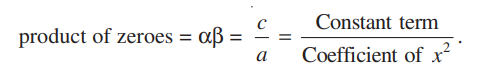2.4 Division Algorithm for Polynomials:- By using the cubic polynomial the section 2.4 has explained the division algorithm for polynomials. As well as some examples have been taken into consideration to illustrate the topic.

Preparation Tips For Polynomials:- Pay attention to each question and try to understand the given terms. Once you master the breaking terms and understand them, you will be able to score higher marks from this chapter.

#### Chapter 3:- Pair of Linear Equations in Two Variables

NCERT Solutions for Class 10 Maths Chapter 3 discusses the equation form ax + by + c = 0. In this linear equation, a,b, and c are the real numbers where a, b equals not zero. In this chapter 3, you will get some Definition of the topics, their formulas, & some examples to solve the equation.

3.1 Introduction: This section recalls what a student has learned in class 8th as well as the section that gives a hint of what students will be able to study through this lesson.
3.2 Pair of Linear Equations in Two Variables:- By recalling the definition of Pair of Linear Equations in Two Variables various example problems are covered in the section 3.2.

3.3 Graphical Method of Solution of a Pair of Linear Equations:- Consistent pair of linear equations and Inconsistent pair of linear equations have been covered in this section. Also, the methods to represent the Solution of a Pair of Linear Equations in graphical methods are given.

3.4 Algebraic Methods of Solving a Pair of Linear Equations:- Since, the graphical presentation of a Pair of Linear Equations will not be the same for all kinds of equations, the algebraic methods have been introduced. This section is focused on teaching students the Algebraic Methods of Solving a Pair of Linear Equations. Various methods are covered in section 3.4: Substitution Method, Elimination Method, Cross - Multiplication Method.

3.5 Equations Reducible to a Pair of Linear Equations in Two Variables:- Section 3.5 deals with such an equation which is not linear but can be reduced to linear equation by making some suitable substitutions.

Preparation Tips For Pair of Linear Equations in Two Variables:- Your basic knowledge in maths will help you to solve the questions with ease. However, you must learn about the formulas and methodology to solve the exercises.

Important formulas:

Below, we have given the general form for a pair of linear equations in two variables x and y:

(i) a3 x + b3 y + c3 = 0

and

(ii) a4 x + b4 y + c4 = 0,

where a3, b3, c3, a4, b4, c4 are real numbers and where a34 + b34 ≠ 0, a44 + b44 ≠ 0.

Interesting fact you may not know: quadratic equations is also known as Equation of Degree. Also, the quadratic equation is not as simple as it sounds. You must understand all terms carefully. The equation you will solve in NCERT Class 10 Maths Chapter 4 will be in the form of degree: ax2 + bx + c = 0, where a, b and c are given values. “a” can’t be 0.

4.1 Introduction:- The intro section tells students how an equation can be called quadratic equations. As per the NCERT Maths book, quadratic polynomials of the form ax2 + bx + c, a ≠ 0. When this equation is being equated with zero, we get a quadratic equation.

4.2 Quadratic Equations:- The section 4.2 deepens the knowledge of Quadratic Equations by explaining various example problems and introducing a few related terms such as the standard form of a quadratic equation.

4.3 Solution of a Quadratic Equation by Factorisation:- Zeroes and roots are the center focus of this subsection where it has been declared that a quadratic equation can have utmost two zeroes and two roots. Along with this, the subtopic provides some simple examples to help students learn how to find Solution of a Quadratic Equation by Factorisation.

4.4 Solution of a Quadratic Equation by Completing the Square:- Method of completing the square has been taught in section 4.4. This is another method of finding the Solution of a Quadratic Equation. Apart from this, the subsection talks about the quadratic formula.

4.5 Nature of Roots:-  The roots of an equation ax2 + bx + c = 0 are given by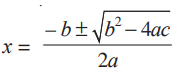. After this, the section 4.5 contains some more detailed intro of nature of roots where the term discriminant has been introduced too. Along with the explanation of these terms, Section 4.5 has several great examples.

4.6 Summary:- Finally, Section 4.6 has the conclusion of all the points which have been explained in NCERT Solutions For Class 10 Maths Chapter 4. There are 6 points the section 4.6 has covered.

Preparation Tips For Quadratic Equations:- In order to properly answer the Quadratic Equations questions, you will have to understand the formulas and ways of using them. We advise you to first go through the solved examples and then refer to the exercises to solve questions. In case you face any kind of difficulty go through the NCERT Class 10 Maths Solutions.

#### Chapter 5:- Arithmetic Progression

You already know this topic, Arithmetic Progression that is also known as “AP”. However, in chapter 5 of Class 10 Maths, you will gather some more in-depth knowledge. The AP has variations such as First Term, General Form, Nth Term, Sum of Nth Term, etc. So, to advance the knowledge in these topics, refer to chapter 5 of class 10th maths.

5.1 Introduction:- We are surrounded with tons of patterns because they are available in our nature such as petals of a sunflower, the holes of a honeycomb, etc. But the patterns are not limited to the nature it is available in our day to day life too. This is what chapter 5 of NCERT Class 10 Maths is trying to teach students through the mathematical term Arithmetic Progression.

5.2 Arithmetic Progressions:- The definition of Arithmetic Progression is very simple: It is a list of numbers in which each term is obtained by adding a fixed number (Fixed Number also known as Common Difference) to the preceding term except the first term. The AP or Arithmetic Progression can be Positive, Negative or Zero. Along with the definition this section 5.2 includes the two more definitions of terms Finite Arithmetic Progression and Infinite Progression.

5.3 nth Term of an AP:- What is the nth term of an AP has been cleared in this section 5.3 along with the definition the formulas has been also mentioned. The nth term an of the AP with first term a and common difference d is given by an = a + (n – 1) d. an is also known as the general term of the AP.

5.4 Sum of First n Terms of an AP:- There is a formula for finding the sum of first n terms of an AP: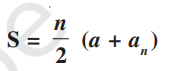. In case, if there are only n terms in an AP then an = l, Here’s the formula for this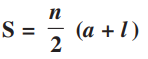. Also for sum of first n positive integers, there is a separate formula: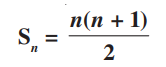. After mentioning all these formulas the section 5.4 contains various example questions with solutions.

5.5 Summary:- After explaining all the terms, the summary section concludes the whole chapters by mentioning the highlighted points. The General form of AP is also mentioned that is a, a + d, a + 2d, a + 3d, . . .

Preparation Tips For Arithmetic Progression:- This is going to be an easy chapter for you to practice. Only, you need is to have a good command over the formulas and definitions given in this chapter.

The general formula of Arithmetic Progression is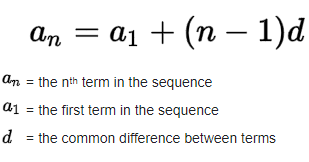Important formulas:

If a1, a2, a3, a4, a5, a6,… are terms of an AP and d is the common difference between each term in the AP, we can write the sequence as; a, a+d, a+2d, a+3d, a+4d, a+5d,….,nth term… where a is the first term of the series. So, the nth term for arithmetic progression is given as;

1. i) nth term = a + (n-1) d

Sum of the first n terms in the Arithmetic Progression;

1. ii) Sn = n/2 [2a + (n-1) d]

#### Chapter 6: - Triangles

One of the most popular Pythagoras Theorem is associated with triangles. Hence, in this chapter 6, you will be able to cover this topic thoroughly. In case you face any difficulty in answering the questions, refer to the NCERT Solutions for Class 10 Maths Chapter 6.

6.1 Introduction:- By giving a short brief of what students have studied in class 9th about triangles and congruent, this intro section briefly explained what students are going to study in this lesson 6 triangles. Here, the topics are not about the congruent but about the similar images when the Two figures have the same shape but not the same size.

6.2 Similar Figures:- Informing students about similar figures, the section covers one important point that is all congruent images can be similar images but all similar images are not necessarily considered congruent. By taking the example of various different geometrical shapes the term Similar Figures have been explained in this section.

6.3 Similarity of Triangles:- A triangle can be called similar if they satisfy the two conditions: (i) the corresponding angles of two triangles are equal (ii) their corresponding sides are in the proportion or same ratio. Along with these, section 6.3 contains two theorems and their proofs, the solved example problems are also there.

6.4 Criteria for Similarity of Triangles:- This section talks about the Criteria for Similarity of Triangles in more detail, as well as a few more theorems have been proved.

6.5 Areas of Similar Triangles:- Inside the NCERT Class 10 Maths Textbook chapter 6, section 6.5 contains the proof of whether the ratio of the similar triangles areas and the ratio of the corresponding sides have any relationship. Along with the theorem 6.6 this section has a few examples and then the exercise 6.4 begins.

6.6 Pythagoras Theorem:- Student’s one of the most popular and favourite Pythagoras Theorem has been covered in this section. Although all 10th standard students are already aware of the Pythagoras Theorem, here in this section, the major focus has been given on proving the theorem using the concept of similarity of triangles.

6.7 Summary:- The summary section includes 13 highlighted points from chapter 6. Along with that there is a note for all the readers. Download NCERT Class 10 Maths Chapter 6 PDF to read what the note is trying to convey the message.

Preparation Tips For Triangles:- Revise your basic knowledge of triangles and then start solving the questions asked in NCERT Class 10th maths chapter 6. To start solving the questions, you can first go for solved examples.

#### Chapter 7: - Coordinate Geometry

When we study geometry using the coordinate points, we call it Coordinate Geometry. With the knowledge of coordinate geometry, we can find distance between two points, dividing lines, midpoints, area of triangle etc.

7.1 Introduction:- The intro section reveals some interesting points such as x-coordinate is called abscissa and y-coordinate is called ordinate. Along with these interesting insights the section 7.1 introduces students what they are going to study in this lesson, Such as how to find the distance between the two points when coordinates are given, and how to find the area formed by three given points.

7.2 Distance Formula:- The distance between Location A to Location B can be calculated using the Pythagoras Theorem. Using some suitable examples the section 7.2 is trying to develop the distance formula: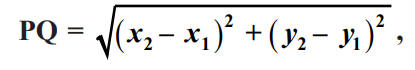After finding out this distance formula, the section has some solved examples for the students to practice.

7.3 Section Formula:- In this section, students will learn the derivation of section formula: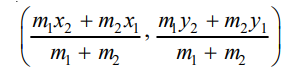. Also, one Special Case has been discussed too when the midpoint divides the line segment into a ratio of 1:1.

7.4 Area of a Triangle:- Area of a triangle can be calculated using the standard formula that is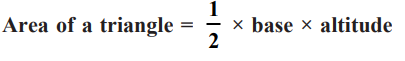, by using Heron’s formula too but the problem may occur when the coordinates of the vertices of a triangle are given. What can be the right method of finding the Area of triangle in such a case has been explained in section 7.4. By explaining about this, one formula has been derived that is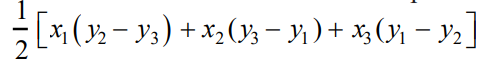.

7.5 Summary:- The Summary section includes all the most important or highlighted terms at a single place; such as formulas, remarks, notes and more.

Preparation Tips For Coordinate Geometry:- You will find this chapter a bit easier in the beginning but as you go further the level of difficulty will rise. Hence, keep in mind to build a strong grip on the basics of geometry and revise the solved questions multiple times. To learn different methods to answer the questions, refer to the NCERT Solutions of class 10 Maths Chapter 7.

#### Chapter 8: - Introduction to Trigonometry

Trigonometry is a vast topic in mathematics, but in class 10 maths, you will get some introductory knowledge of trigonometry. Chapter 8, trigonometry enables you to gather the knowledge of trigonometric Ratios from 0 to 90 degrees. This lesson also allows you to learn to find the Relation between Trigonometric Ratios, and more.

8.1 Introduction:- Before this class, the students are already familiar with the topics of trigonometry, however, in this section the Trigonometry has been discussed in detail such as about the ratios, ratio of some specific angles, trigonometric Identities, etc.

8.2 Trigonometric Ratios:- The trigonometric ratios of an acute angle in a right triangle indicate the relationship between the angle and the length of its sides. But this is a short definition of the trigonometric ratios apart from this there are many relevant or linked terms which have been explained in section 8.2.

8.3 Trigonometric Ratios of Some Specific Angles:- The section 8.3 includes the methods of finding the values of the trigonometric ratios for the specific angles such as 30°, 45°,
60° and 90° including Zero degree.

8.4 Trigonometric Ratios of Complementary Angles:- When the sum of two angles are equal to 90 degrees it is said to be a complementary angle. Along with definition of complementary angles the section has covered the methods to find out the Trigonometric Ratios for the Complementary Angles.

sin (90° – A) = cos A, cos (90° – A) = sin A,
tan (90° – A) = cot A, cot (90° – A) = tan A,
sec (90° – A) = cosec A, cosec (90° – A) = sec A

8.5 Trigonometric Identities:- This section has covered the proof of one trigonometric identity and after that the other Trigonometric Identities have been proved. For example the example 15 in the NCERT Class 10 Maths Book Chapter 8 contains the question to proof the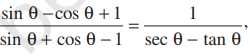using the identity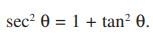.

8.6 Summary:- All the key takeaways of the lesson have been mentioned in the summary section.

Preparation Tips For Introduction to Trigonometry:- Remember all the trigonometric Ratios from 0 to 90 degrees. And before solving a question, read them multiple times and try to break them in chunks. It will make the task easier for you to solve any kind of question.

Trigonometry Table:

 Angle 0° 30° 45° 60° 90° Sinθ 0 1/2 1/√2 √3/2 1 Cosθ 1 √3/2 1/√2 ½ 0 Tanθ 0 1/√3 1 √3 Undefined Cotθ Undefined √3 1 1/√3 0 Secθ 1 2/√3 √2 2 Undefined Cosecθ Undefined 2 √2 2/√3 1#### Chapter 9: - Some Applications of Trigonometry

Trigonometry is used widely in finding the distance between landmarks, astronomy, for satellite navigations and more. Hence, in this NCERT Class 10 Maths Solutions Chapter 9, you will learn how to use trigonometry to solve real life problems, as well as to solve the exercises questions.

9.1 Introduction:- The trigonometry has uses in daily life and it is used by our ancients and we use this subject till the date especially in astronomy. So, this lesson has covered the uses of Trigonometry in daily life.

9.2 Heights and Distances:- Line of sight, angle of elevation, angle of depression, and etc. terms have been discussed in this section.

9.3 Summary:- The summary section contains all the key points of this lesson for the revision purpose.

Preparation Tips For Some Applications of Trigonometry:- Just stick with the basic knowledge and the formulas. NCERT 10th Maths Chapter 9 is all about implementing your knowledge of trigonometry. So, solve the asked questions and implement the given NCERT solutions to tackle difficult questions.

#### Chapter 10: - Some Applications of Circles

In Class 10 Maths Chapter 10, you will learn the definition of circle, its terminology, and the methods of solving the questions related to the Circles. To answer the asked questions in this chapter, you will need to build a strong grip on the basics knowledge of circles, and all of its properties.

10.1 Introduction: The intro section contains a bit brief of the topic circle and about chord, segment, sector, arc etc. However, the major focus of this lesson is to teach students about examining the different scenarios when a circle and a line
are given in a plane.

10.2 Tangent to a Circle: The intro to tangent has been covered in the section 10.1 however, in this section 2 tasks/activities have been performed to understand the existence of the tangent to a circle at a point.

10.3 Number of Tangents from a Point on a Circle:- Theorem 10.2 and a few activities have been done in this section to understand the number of tangents from a point on a circle.

10.4 Summary:- Whatever the lesson has discussed across this lesson, the summary section includes all the key points from them.

Preparation Tips For Some Applications of Circles:- First of all solve the lower level of questions related to circles then go for difficult one. If possible then try to solve the questions of circles from the previous class (9th or 8th).

#### Chapter 11: - Construction

The aim of chapter 11, construction is to help you learn how to construct various diagrams. With the help of this lesson, you will learn the methods of Drawing Tangents to a Circle, and more related questions. The NCERT Solutions for Class 10 Maths Chapter 11 covers all the problems in depth.

11.1 Introduction:- The brief of construction that has been taught to the students in earlier class as well as what topics they will learn in this chapter has been covered in the Intro section of NCERT Class 10 maths solutions chapter 11.

11.2 Division of a Line Segment:- Steps of Construction, alternative methods and various examples have been given in section 11.2 to make students understand the methods to divide the line segment in a given ratio.

11.3 Construction of Tangents to a Circle: The section 11.3 helps students understand how to draw a tangent to a circle from a point outside it.

11.4 Summary:- 3 important points have been recalled in this section 11.4 about the constructions.

Preparation Tips For Construction:- Many students don’t understand the questions properly but they try to answer them. Doing so will not be helpful in solving the questions of construction. Refer to the NCERT Solutions to understand the questions in step by step methods.

#### Chapter 12: - Areas Related to Circles

A circle contains circumference, segment, sector and angle etc. so to learn about them refer to the chapter 12 of NCERT Class 10 Maths Solutions. It will help you to solve all the Areas Related to Circles questions with ease.

12.1 Introduction:- After the brief of how familiar the students are with the circle, the introduction section indicates what students can expect from this lesson such as how to find the areas of some combinations of plane figures including circles or their parts.

12.2 Perimeter and Area of a Circle — A Review:- The section 12.2 includes a short definition of Perimeter and circumference. Along with the definition their formulas have been discussed too. Basically, a review of Perimeter and Area of a Circle has been mentioned by using some good examples.

12.3 Areas of Sector and Segment of a Circle:-  Minor segment, Major Segment, Area of the sector of angle, length of an arc of a sector of angle θ, etc. are the center focus of this section. These terms have been explained using relevant examples. Apart from these, a student can access some of the important formulas here:

• Area of the sector of angle θ =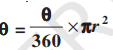• Length of an arc of a sector of angle θ=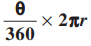12.4 Areas of Combinations of Plane Figures:- After learning the methods of finding the area of different figures, this section discusses the process to calculate the areas of some combinations of plane figures.

12.5 Summary:- 5 major points are mentioned in this section from the whole lesson. Almost all are formulas.

Preparation Tips For Areas Related to Circles:- Area of a circle is known as πr2, this is one of the most popular terms in academic maths. However, to solve the questions of Areas Related to Circles you may have to apply different methodology, thus refer to the solution book available here on this website.

Important formulas:

• circumference = 2πr
• area of the circle = πr 2
• Area of the sector of angle θ = (θ/360) × π r2
• Length of an arc of a sector of angle θ = (θ/360) × 2 π r where r is the radius of the circle

#### Chapter 13: - Surface Areas and Volumes

You already know how to find the Surface Areas and Volumes of cube, cuboid, cone, cylinder, and etc; but you used to solve some basic level questions in previous classes. Here in this 10th standard, you will have to answer some complex questions that are a combination of different solid shapes.

13.1 Introduction:- Every day we all come across many solid shapes such as cuboids, cones, cylinders, etc. Thus, in this lesson the learners have been taught the methods  to find surface areas and volumes of such objects.

13.2 Surface Area of a Combination of Solids:- We often come across the combination of solid shapes for example the given figure 13.2, where a combination of cylinder and hemispheres can be seen. Now, section 12.2 explains to students about the methods to find the surface area of a combination of solids for such figures or objects.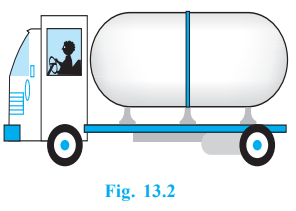13.3 Volume of a Combination of Solids:- Through some good examples the section 13.3 helps students to learn how to find the Volume of a Combination of Solids. Also, the section defines the differences between calculating the Volume and Surface area of a solid.

13.4 Conversion of Solid from One Shape to Another: Solid shapes are converted from one shape to another, the good example of this conversion is when a candle burns. Hence, the center focus of this section is to teach students how to calculate area, size, volume, etc. of a converted solid shape and its original shapes.

13.5 Frustum of a Cone:- Frustum is a Latin word the original meaning of this word is “piece cut off”. Henec, in this section the students will study about the cut piece of a cone which is also known as Frustum of a Cone.

Figure 13.20 explain this in simple way by illustrating them like this: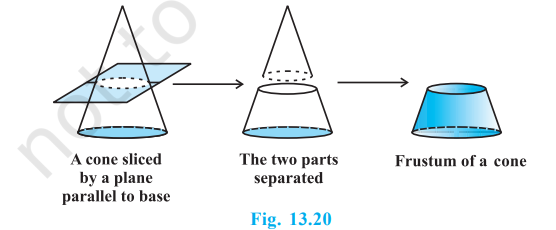. Along with these, the Volume of the frustum of the cone,  curved surface area, total surface area of the cone, etc. have been covered.

13.6 Summary:- In this section, 4 points are mentioned that indicate what students have studied so far in this lesson.

Preparation Tips For Surface Areas and Volumes:- Basic knowledge is enough, but make sure you have a good command over all the formulas.

Important formulas:

• TSA of new solid = CSA of one hemisphere + CSA of cylinder + CSA of another hemisphere
• Diameter of sphere = 2r
• Surface area of sphere = 4 π r2
• Volume of Sphere = 4/3 π r3
• Curved surface area of Cylinder = 2 πrh
• Area of two circular bases = 2 πr2
• Total surface area of Cylinder = Circumference of Cylinder + Curved surface area of Cylinder = 2 πrh + 2 πr2
• Volume of Cylinder = π r2 h
• Slant height of cone = l = √(r2 + h2)
• Curved surface area of cone = πrl
• Total surface area of cone = πr (l + r)
• Volume of cone = ⅓ π r2 h
• Perimeter of cuboid = 4(l + b +h)
• Length of the longest diagonal of a cuboid = √(l2 + b2 + h2)
• Total surface area of cuboid = 2(l×b + b×h + l×h)
• Volume of Cuboid = l × b × h

#### Chapter 14: - Statistics

One of the most important chapters, if you want to build your future career in data analyst, AI, & Machine learning technology. However, 10th standard statistics will not make you a data analyst but it gives you the basic knowledge of the topics and how to prepare the data and use them. NCERT Class 10 Maths Chapter 14, Statistic covers various methods, Types of Data, Types of quantitative data, Types of Statistics, and their examples.

14.1 Introduction:- The chapter 14 is an extended version of mean, median and mode. This is what the introduction section has mentioned. Apart from these, a few important points have been discussed.

14.2 Mean of Grouped Data:- Along with the definition of mean of grouped data a formula that is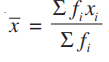has been mentioned in this section. Along with these things, how to use this formula has been instructed to the students by using some appropriate examples. The Assumed Mean Method and Step-deviation have been also discussed in this section.

14.3 Mode of Grouped Data:- value of the observation having the maximum frequency is known as Mode of Grouped Data. Since this has been already discussed with the students in class 9th here, the learners will learn the ways of obtaining a mode of grouped data. Modal class. The formula of mode is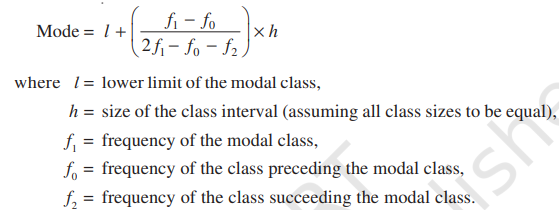14.4 Median of Grouped Data:- Recalling the ways of finding the median of ungrouped data, the section 14.4 teaches students how to find the Median of Grouped Data. Also the  empirical relationship between the three measures of central tendency has been covered, that is 3 Median = Mode + 2 Mean.

14.5 Graphical Representation of Cumulative Frequency Distribution:- This section includes methods to represent the Cumulative Frequency Distribution in graphical ways. For example the given figure: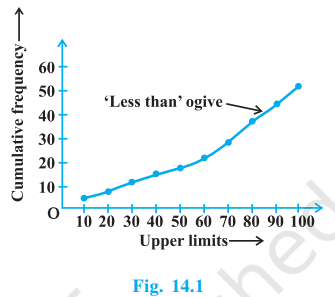.
The definition of Cumulative Frequency Distribution has been also given in this section.

14.6 Summary:- Summarises all the important points of the chapter 14 Statistics. For example the mean for grouped data can be found by three methods: (i) Direct method, (ii) Assumed mean method, (iii) Step deviation method.

Preparation Tips For Statistics:- To solve the questions asked in 10th maths chapter 14, you should have knowledge about linear algebra, stochastic analysis, differential equations etc.

Important formulas:

The mean of the grouped data can be found by 3 methods:

• Direct Method: x̅ =
•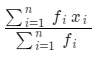, where ∑fi xi is the sum of observations from value i = 1 to n And ∑fi is the number of observations from value i = 1 to n
• Assumed mean method: x̅ ==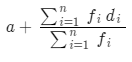• Step deviation method: x̅ =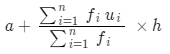The mode of grouped data:

Mode =The median for grouped data:

Median =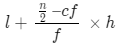#### Chapter 15: - Probability

Possibility in the occurrence of a random event is known as Probability. The knowledge of probability mainly helps in predicting some events.

In this chapter, you will learn Definition, Formula, and several methods of predicting some events. You may need to use some previously studied knowledge into it, to solve.

15.1 Introduction: Along with the brief of what a student has studied in their class 9th maths about Probability, the section 15.1 talks about what students will study in this lesson:  classical Probability or theoretical probability of an event.

15.2 Probability — A Theoretical Approach:- In this section, the method of writing a theoretical probability has been developed when all outcomes of an event is equally likely then, it will written as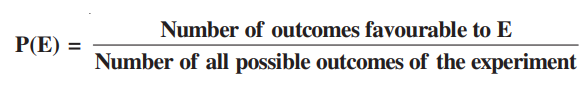. Impossible events, Sure events and such more interesting topic’s definitions are given.

15.3 Summary:- Summary section contains the most important points from the lesson such as the difference between experimental probability and theoretical probability?, How to write Classical or Theoretical Probability? etc.

Preparation Tips For Probability:- Your common sense and some given formulae is quite enough to solve the probability questions.

Important formulas:

1. The theoretical probability (also called classical probability) of an event E, written as P(E), is defined as: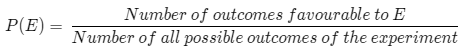where we assume that the outcomes of the experiment are equally likely.

1. The probability of a sure event (or certain event) is 1.
2. The probability of an impossible event is 0.
3. The probability of an event E is a number P(E) such that 0 ≤ P (E) ≤ 1
4. An event having only one outcome is called an elementary event. The sum of the probabilities of all the elementary events of an experiment is 1.

### Importance of Learning Mathematics from Class 10 Maths NCERT SolutionsIt is important to learn CBSE NCERT Maths Solutions of Class 10. Because, it helps you to be more informative and develop problem solving skills. Also, it is crucial to score higher in the board examination.

Apart from the above importance, below are some of the points that describe the importance of learning 10th maths from NCERT Solutions of Class 10 Maths.

It Enhances The Basic Level Knowledge:- Basics are core, no matter which kind of problem you are solving, if your basics are stronger, you will figure out ways to solve them. Therefore, NCERT Solutions of 10th maths are considered important because it helps you to enhance your basic level knowledge.

Helps You To Cover Entire 10th Maths Questions:- Covering all the maths questions are extremely important because questions are asked in the board exams. Question papers are prepared by referring to the NCERT Class 10th maths and that is why the NCERT Solutions of maths are important because it includes all the chapter wise solutions. All solutions are prepared by subject matter experts keeping in mind the exam pattern and understanding level of students.

Gives Boost In Confidence Level:- Unable to solve a question, lowers the confidence level of a student. Therefore, the NCERT Solutions are too good for such a case. With the help of these solutions, students can solve all types of NCERT 10th maths questions regardless of its difficulty level. Doing so enables the students to boost their confidence level.

### What is the Exam pattern of CBSE Class 10 Maths in 2022-23?Exam pattern of CBSE Class 10 Maths in 2022-23 is as below mentioned:

• Tests (PT) for 10 marks, Notebook Submissions for 5 marks and Subject Activities for 5 marks each.
• The theory paper of Maths is for 80 marks.
• The duration of the exam is 3 hours.
• The minimum passing percentage in maths is 33%.

#### NCERT Class 10th Mathematics Chapter-wise Marks Weightage

 Unit Title Weightage 1 Number System 06 2 Algebra 20 3 Co-ordinate Geometry 06 4 Geometry 15 5 Trigonometry 12 6 Mensuration 10 7 Statistics And Probability 11 Total Marks 80

#### CBSE Class 10 Maths Question Paper Design for Academic Year 2021-22:-

 Sr. No Type of Questions Total Marks Weightage 1 Memory: Terms, answers, basic concepts and recalling of facts. Understanding: Compare, organize, interpret, translate, describe and stating main ideas. 43 54 2 Apply: Solving problems by applying the knowledge, facts, theorems and rules in different ways. 19 24 3 Analyse: Analyse the information provided and make inferences.  Evaluate: Opine about the validity of quality of work and validity of ideas. Create: Creating alternative solutions to the given problem and grouping the elements. 18 22 Total 80 100

### How NCERT Solutions for Class 10 Maths are Helpful for Board Exams?NCERT Class 10 Maths Solutions are very helpful for the board exams because the teachers who prepare the board questions, pick the problems from the prescribed NCERT Class 10 Maths Book.

So, our subject matter experts used the same books and solved all the questions in step by step methods. Due to this, students can easily clear their doubts and prepare for the exam with ease.

The solutions not only help in clearing the concepts but enable the students to clear all their doubts.

Solutions also help students to learn and develop the creative and rational thinking ability that is eventually used during the board exam.

#### Important Tips for Scoring Well in the Board Exams

Maintain Regularity: Exam preparation couldn’t be better, if you are not regular in your study. It is important for you to maintain regularity while preparing for the Class 10 Board exam. To maintain the regularity you must study daily, once you come back from the school, try to invest a good time in revising all the topics you have studied in the classroom. Doing this will help you to retain your learning for a longer period and allow you to score better marks in the board exams.

Practice A lot: Practice is key to exam preparation, you must go through the whole chapter wise NCERT Solutions, Previous year question papers, & sample papers. Also, go for the NCERT Class 10th maths book and solve all the given examples one by one. In case you are stuck somewhere in the middle, take help of the given solutions.

Clear All Concepts & Confusion: No question should remain unclear, all questions and topics must be clear by you, before appearing in the board exams. Whether you ask your teacher or take online study materials help, make sure you don’t have any confusion in topics/concepts.

Give Importance To Syllabus: Before diving into practice the questions, go through the whole syllabus and understand the topics and their subtopics, their weightage also matters. Thus, give importance to the syllabus and prepare well for the exam.

Identify Your Strength & Weakness: You should have a good grasp on each topic, if you don’t have any idea where your strengths and weaknesses are then refer to the Previous Year question papers & sample question papers to Identify Your Strength & Weakness in the subject. Once you are done with it, pay close attention to weak areas to improve them.

Follow A Proper Time Table: Discipline in study is very important, hence prepare a proper time table and follow them honestly. Using a time table will aid you to stay motivated and complete your syllabus as planned. It is also good to use, because you shouldn’t leave any chapter undone. So, Follow A Proper Time Table and set a daily goal to achieve them as well as to complete the syllabus.

###Best Reference Books for Class 10 Maths, Science and SST are given below

RD Sharma Class 10th Mathematics Textbook:- RD Sharma Class 10 Maths Textbook is one of the renowned books among the students. It is student-friendly, it has a variety of questions along with higher explanations and solutions. All are extremely detailed and given in the use of simple language. The RD Sharma books can help you to cover the entire syllabus of 10th. On Selfstudys you will be able to get the Class 10 Maths solutions of Sharma’s book that are prepared by maths experts.

RS Aggarwal Class 10 Mathematics Textbook:- RS Aggarwal Class 10 Maths Textbook is considered to have a low level of difficulty. If a student has completed the RD Sharma book then solving the RS Aggarwal textbooks will not be a big deal. It is also one of the famous books but with a moderate level of questions.

If you are a beginner and want to start your maths learning, the NCERT and RS Aggarwal maths will be highly suggestible.

Lakhmir Singh and Manjith Kaur:- Lakhmir Singh and Manjith Kaur book is a reference book for class 10th Physics, Chemistry and Biology. The chapters are explained in detail but they are very precise. You will be able to easily grasp the concepts from this book. Furthermore, if you face any difficulty in solving the questions of these books, then feel free to download the solutions we have provided here on this website.

#### Total number of chapters are there in Class 10 Maths NCERT Solutions

There are total number of chapters 15 in CBSE Class 10 NCERT Maths books as per session 2022-23. The chapters names are Chapter 1- Real Numbers, Chapter 2 - Polynomials, Chapter 3 - Pair of Linear Equations in Two Variables, Chapter 4 - Quadratic Equations, Chapter 5 - Arithmetic Progressions, Chapter 6 - Triangles, Chapter 7 - Coordinate Geometry, Chapter 8 - Introduction to Trigonometry, Chapter 9 - Some Applications of Trigonometry, Chapter 10 - Circles, Chapter 11 - Constructions, Chapter 12 - Areas related to Circles, Chapter 13 - Surface Areas and Volumes, Chapter 14 - Statistics and Chapter 15 - Probability.

####FAQs

You can get the NCERT Solutions for Class 10 Maths PDF on Selfstudys. The solutions are prepared by the maths experts keeping in mind the understanding level of students. The main purpose of creating solutions is to help students learn the complex concepts without facing any difficulty. Students can also cross check their answers with the help of solutions.

In order to master your 10 class maths of CBSE, you need to focus on finishing your NCERT syllabus. This involves solving all the questions and the examples thoroughly. To help you with this, Selfstudys has brought the NCERT Solutions for Class 10 Maths in PDF format. You can use these as a guide for finishing the NCERT exercises of mathematics. All the solutions are prepared and given in the step by step manner.

The advantages of NCERT Solutions for Class 10 Maths can be numerous. The first and foremost advantage of maths solutions is that it guides the students to answer the NCERT Maths exercise questions easily. Helps in cross checking your given answers, and it aids in the board exam preparations.

Yes, NCERT Solutions for Class 10 Maths help you to solve the problems faster and easier but you must have a thorough understanding of class 10th syllabus. Mathematics is a great subject that can help you to score upto 100% marks. But a few are able to do this, because most students don’t study well and are unable to understand the maths concepts precisely. However, the NCERT Solutions for class 10 maths can help you to improve the logical thinking and analytical skills among students.

The concepts that are explained in the solutions of NCERT Class 10 Maths Textbook are many. In CBSE 10th Class there are 15 chapters in mathematics and all of them have been explained in the Selfstudys solutions of NCERT Class 10th Maths. It is extremely helpful and prepared by the maths experts.

Best Reference Books for Class 10 Maths are given below

Yes, for the Class 10 board exam, the NCERT maths textbook is quite enough. However, you must go through all solved previous year’s papers and sample papers to boost your preparation level.

No doubt, enormous practice of questions will help you to score higher marks in class 10th board exam of maths. But, you must pay attention to the exam pattern, marking schemes and all the important questions.

NCERT Class 10 Maths Book contains a total of 15 chapters, which can be used to cover the syllabus and to learn a variety of interesting topics.

Selfstudys website is the best website for NCERT Maths Solutions of class 10 - all students can trust on this platform. Each solution has been prepared by the subject matter experts in simple language to guide students to understand the complex questions with ease.

NCERT Class 10 Maths Solutions are prepared by experts referring to the syllabus to help you answer the complex questions with ease. The aim of the solution is to assist you to clear your confusions in the topics and answer all the complex questions. With the help of it, you can strengthen your understanding in the subject as well as, you will be able to score higher marks.

Related News
See All×
Open Now